# Sick days

In Canada, there are typically 261 working days per year. If there is a 4.9% chance that an employee takes a sick day. ..
what is the probability an employee will use 17 OR MORE sick days in a year?

Correct result:

p =  0.0929

#### Solution:We would be pleased if you find an error in the word problem, spelling mistakes, or inaccuracies and send it to us. Thank you!Tips to related online calculators
Looking for a statistical calculator?
Would you like to compute count of combinations?

## Next similar math problems:

• Component fail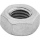There is a 90 percent chance that a particular type of component will perform adequately under high temperature conditions. If the device involved has four such components, determine the probability that the device is inoperable because exactly one of the
• RecordsRecords indicate 90% error-free. If 8 records are randomly selected, what is the probability that at least 2 records have no errors?
• All use computerIt is reported that 72% of working women use computers at work. Choose 3 women at random, find the probability that all 3 women use a computer in their jobs.
• Boys and girlsThere are 11 boys and 18 girls in the classroom. Three pupils will answer. What is the probability that two boys will be among them?
• Family94 boys are born per 100 girls. Determine the probability that there are two boys in a randomly selected family with three children.
• Six questions testThere are six questions in the test. There are 3 answers to each - only one is correct. In order for a student to take the exam, at least four questions must be answered correctly. Alan didn't learn at all, so he circled the answers only by guessing. What
• SalesFrom statistics of sales goods, item A buy 51% of people and item B buys 59% of people. What is the probability that from 10 people buy 2 item A and 8 item B?
• Playing cards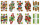From 32 playing cards containing 8 red cards, we choose 4 cards. What is the probability that just 2 will be red?
• Internet anywhere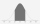In school, 60% of pupils have access to the internet at home. A group of 8 students is chosen at random. Find the probability that a) exactly 5 have access to the internet. b) At least 6 students have access to the internet
• Left handedIt is known that 25% of the population is left-handed. What is the probability that there is a maximum of three left-handers at a seminar where there are 30 participants?
• TestThe teacher prepared a test with ten questions. The student has the option to choose one correct answer from the four (A, B, C, D). The student did not get a written exam at all. What is the probability that: a) He answers half correctly. b) He answers al
• DiceWe throw five times the dice. What is the probability that six fits exactly twice?
• DeficienciesDuring the hygienic inspection in 2000 mass caterers, deficiencies were found in 300 establishments. What is the probability that deficiencies in a maximum of 3 devices will be found during the inspection of 10 devices?
• Hazard game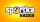In the Sportka hazard game, 6 numbers out of 49 are drawn. What is the probability that we will win: a) second prize (we guess 5 numbers correctly) b) the third prize (we guess 4 numbers correctly)?
• Families 2There are 729 families having 6 children each. The probability of a girl is 1/3 and the probability of a boy is 2/3. Find the the number of families having 2 girls and 4 boys.
• Probability of failures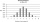In certain productions, the probability of failures is 0.01. Calculate the probability that there will be more than 1 failure among the 100 selected products if we return the selected products to the file after the check.
• The testThe test contains four questions, and there are five different answers to each of them, of which only one is correct, the others are incorrect. What is the probability that a student who does not know the answer to any question will guess the right answer